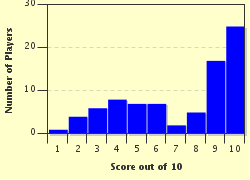FREE! Click here to Join FunTrivia. Thousands of games, quizzes, and lots more!# Korean Numbers Trivia Quiz

### Here's an easy quiz about Korean numbers to those who are already familiar with the Sino-Korean numbers and Native Korean numbers.

A multiple-choice quiz by Sari73. Estimated time: 3 mins.

Author
Time
3 mins
Type
Multiple Choice
Quiz #
367,113
Updated
Dec 03 21
# Qns
10
Difficulty
Average
Avg Score
8 / 10
Plays
632
Last 3 plays: Guest 216 (8/10), Guest 173 (10/10), Guest 112 (0/10).
-
Question 1 of 10
1. Complete the equation of five plus four into Sino-Korean numbers. Hint

#### NEXT>

Question 2 of 10
2. How would you say 45 in Sino-Korean? Hint

#### NEXT>

Question 3 of 10
3. How would you complete the equation 9 x 12 in Sino-Korean? Hint

#### NEXT>

Question 4 of 10
4. How would you complete the equation 100 x 10 in Sino-Korean? Hint

#### NEXT>

Question 5 of 10
5. How would you say 10,000 in Korean? Hint

#### NEXT>

Question 6 of 10
6. "Pal Ship Sa" is the numeral 84 in Sino-Korean.

#### NEXT>

Question 7 of 10
7. How would you solve the equation 9 + 5 in Native Korean numerals? Hint

#### NEXT>

Question 8 of 10
8. How would you solve the equation 9 + 1 in Native Korean numerals? Hint

#### NEXT>

Question 9 of 10
9. How would you complete the equation 1000 + 4? Hint

#### NEXT>

Question 10 of 10
10. The number 1999 is pronounced 'Chun Gu Beck Gu Ship Gu' in Sino-Korean.

 (Optional) Create a Free FunTrivia ID to save the points you are about to earn:Select a User ID:Choose a Password:Your Email:

Most Recent Scores
Nov 28 2023 : Guest 216: 8/10
Nov 27 2023 : Guest 173: 10/10
Nov 20 2023 : Guest 112: 0/10
Nov 19 2023 : Guest 76: 10/10
Nov 19 2023 : Guest 49: 10/10
Nov 14 2023 : Guest 183: 10/10
Nov 08 2023 : Guest 94: 6/10
Nov 07 2023 : Guest 203: 10/10
Nov 06 2023 : Guest 73: 8/10

Score DistributionQuiz Answer Key and Fun Facts
1. Complete the equation of five plus four into Sino-Korean numbers.

5 + 4 = 9, "Gu", the answer would be number 9. "Ship" = 10, "Pal" = 8, "Chil" = 7. The Sino-Korean number system is significant for dates, money, addresses, and phone numbers.
2. How would you say 45 in Sino-Korean?

45 is "Sa Ship Oh" in Sino-Korean numbers. "Oh Ship Sa" is 54. "Ship Oh" is 15, "Ship Gu" is 19.
3. How would you complete the equation 9 x 12 in Sino-Korean?

The equation 9 x 12 = 108, or "Beck pal" in Sino-Korean.
"Beck" = 100, "Pal" = 8, "Ship" = 10, "Sa" = 4.
4. How would you complete the equation 100 x 10 in Sino-Korean?

The equation 100 x 10 = 1000, or "Chun." "Beck" = 100. "Sa" = 4.
"Chun Beck" would be 100,000. "Chun Sa" would be 1004.
5. How would you say 10,000 in Korean?

"Man" is 10,000, "Chun Beck" is 100,000, "Chun Sam" is 1003, "Chun Oh" is 1005.
Most Korean currency is in the form of "Man Won."
6. "Pal Ship Sa" is the numeral 84 in Sino-Korean.

"Pal ship" is 80, "Sa" is 4 in Korean numbers.
"Pal Ship Sa" = 84.
1984 would be "Chun Gu Beck Pal Ship Sa."
7. How would you solve the equation 9 + 5 in Native Korean numerals?

The answer 14 is "Yul Net", in Native Korean numbers, the system to determine age or to determine number of items (1-99).
"Yul Set" is 13, "Yul Daseot" is 15, "Sue Mul" is 20.
8. How would you solve the equation 9 + 1 in Native Korean numerals?

"Yul" is 10 in Native Korean numbers.
"Hana" is one, "Aheop" is nine, "Yul Hana" is eleven.
The Native Korean numeral system is said to determine age.
9. How would you complete the equation 1000 + 4?